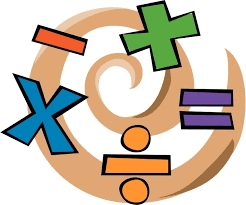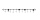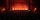# Equivalent fractions 2

Write the equivalent multiplication expression.

2 1/6÷3/4

x =  2.8889

### Step-by-step explanation:Did you find an error or inaccuracy? Feel free to write us. Thank you!Tips to related online calculators
Need help to calculate sum, simplify or multiply fractions? Try our fraction calculator.

## Related math problems and questions:

• Fractions and mixed numerals(a) Convert the following mixed numbers to improper fractions. i. 3 5/8 ii. 7 7/6 (b) Convert the following improper fraction to mixed number. i. 13/4 ii. 78/5 (c) Simplify these fractions to their lowest terms. i. 36/42 ii. 27/45 2. evaluate following ex
• Divide 11Divide the product of 4 and 5/8 by 1 1/2. Write your answer as a mixed number.
• Numbers divisionWith what number should be divided mixed number 2 3/4 to get 11/12?
• Adding mixed numerals3 3/4 + 2 3/5 + 5 1/2 Show your solution.
• Simplify 3Simplify mixed numerals expression: 8 1/4- 3 2/5 - (2 1/3 - 1/4) Show your solution.
• Between two mixedWhat is the rational number between 2 1/4 and 2 4/5?
• Addition of mixed numeralsAdd two mixed fractions: 2 4/6 + 1 3/6
• A large 2A large popcorn bag holds four times as much as a small popcorn bag at the end of the party 3 1/3 small bags and 2 1/4 large bags left. How many small bags with the leftover popcorn fill?
• Difference mixed fractionsWhat is the difference between 4 2/3 and 3 1/6?
• PizzasBilly ate 1 1/4 pizzas and John ate 1 2/3 pizzas. How much more pizza did John eat than Billy?
• EquationSolve equation and check the result: 1.4x - 3/2 + x - 9,8 = x + 0,4/3 - 7 + 1,6/6
• Max vs SharonMax is 1 1/2 times as tall as Sharon. Alan is 4 1/3 feet tall. If Alan is 6 inches taller than Sharon, how tall is Max?
• Quotient 3If the quotient of 8/13 and 2 is subtracted from the product of 1 3/4 and 8/21, what is the difference?
• EvaluateThe division of numbers 6 and 3 increase by-product of the numbers 115 and 0.1
• Adding mixed 42 and 1/8th plus 1 and 1/3rd =
• Fraction of a NumberIf 1/2 of 1/3 of 1/4 of 1/5 of a number is 2.5. what is the number?
• Evaluate expressionEvaluate expression using BODMAS rule: 1 1/4+1 1/5÷3/5-5/8